# MA.912.F.1.1Export Print
Given an equation or graph that defines a function, determine the function type. Given an input-output table, determine a function type that could represent it.

### Clarifications

Clarification 1: Within the Algebra 1 course, functions represented as tables are limited to linear, quadratic and exponential.

Clarification 2: Within the Algebra 1 course, functions represented as equations or graphs are limited to vertical or horizontal translations or reflections over the x-axis of the following parent functions: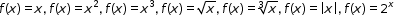and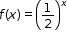.

General Information
Subject Area: Mathematics (B.E.S.T.)
Strand: Functions
Status: State Board Approved

## Benchmark Instructional Guide

### Terms from the K-12 Glossary

• Exponential Function
• Function
• Linear Function

### Vertical Alignment

Previous Benchmarks

Next Benchmarks

### Purpose and Instructional Strategies

In grade 8, students identified the domain and range of a relation and determined whether it is a function or not. In Algebra I, students classify function types limited to simple linear, quadratic, cubic, square root, cube root, absolute value and exponential functions. In later courses, students will classify other function types.
• The purpose of this benchmark is to lay the groundwork for students to be able to choose appropriate functions to model real-world data.
• Instruction includes the connection of the graph to its parent function. See Clarification 1 for specifics of the Algebra I course.
• Students will work extensively with linear, quadratic and exponential models in the Algebra I course. Strong attention should be given to the other function types so that students can build familiarity with them. As new function types are introduced, take time to allow students to produce a rough graph of the parent function from a table of values they develop. Lead student discussion to build connections with why these function types produce their corresponding graphs (MTR.4.1).
• Instruction develops the understanding that if given a table of values, unless stated, one cannot absolutely determine the function type, but state which function the table of values could represent.
• For example, if given the function $y$ = |$x$| and only positive values were given in a table, one could say that table of values could represent a linear or absolute value function.

### Common Misconceptions or Errors

• Some students may miscalculate first and second differences that deal with negative values, especially if they perform them mentally. In these cases, have students quickly write out the subtraction expression (i.e., −14 – (−2)) so they can see that they are subtracting a negative value and should convert it to adding a positive value.

### Strategies to Support Tiered Instruction

• Teacher provides opportunities to write out subtraction sentences next to each line of the table when determining first and second differences.
• Instruction is provided to determine the type of function the graph represents. Knowledge on the end behavior of different types of functions may provide students with additional information to identify different types of functions. The teacher co-creates an anchor chart showing different types of functions and their end behavior
• Teacher provides methods for calculating and/or interpreting the first and second differences given a table of values.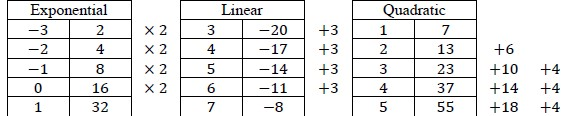• Instruction includes opportunities to use graphing software to graph parent functions of different equations (i.e., square root, cubic, absolute value, etc.).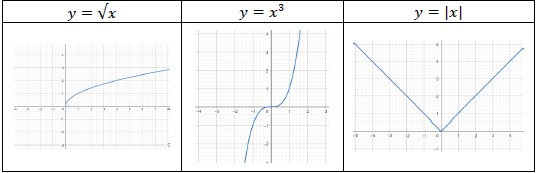• Given the graphs below, identify each type function it represents. Justify your answer.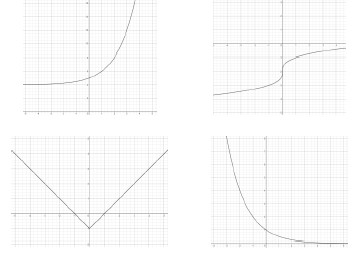### Instructional Items

Instructional Item 1
• Given the table below, determine the function type that could represent it.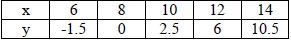*The strategies, tasks and items included in the B1G-M are examples and should not be considered comprehensive.

## Related Courses

This benchmark is part of these courses.
1200310: Algebra 1 (Specifically in versions: 2014 - 2015, 2015 - 2022, 2022 and beyond (current))
1200320: Algebra 1 Honors (Specifically in versions: 2014 - 2015, 2015 - 2022, 2022 and beyond (current))
1200330: Algebra 2 (Specifically in versions: 2014 - 2015, 2015 - 2022, 2022 and beyond (current))
1200340: Algebra 2 Honors (Specifically in versions: 2014 - 2015, 2015 - 2022, 2022 and beyond (current))
1200370: Algebra 1-A (Specifically in versions: 2014 - 2015, 2015 - 2022, 2022 and beyond (current))
1200380: Algebra 1-B (Specifically in versions: 2014 - 2015, 2015 - 2022, 2022 and beyond (current))
1200400: Foundational Skills in Mathematics 9-12 (Specifically in versions: 2014 - 2015, 2015 - 2022, 2022 and beyond (current))
7912080: Access Algebra 1A (Specifically in versions: 2014 - 2015, 2015 - 2018, 2018 - 2019, 2019 - 2022, 2022 and beyond (current))
7912090: Access Algebra 1B (Specifically in versions: 2014 - 2015, 2015 - 2018, 2018 - 2019, 2019 - 2022, 2022 and beyond (current))
1200315: Algebra 1 for Credit Recovery (Specifically in versions: 2014 - 2015, 2015 - 2022, 2022 and beyond (current))
1200375: Algebra 1-A for Credit Recovery (Specifically in versions: 2014 - 2015, 2015 - 2022, 2022 and beyond (current))
1200385: Algebra 1-B for Credit Recovery (Specifically in versions: 2014 - 2015, 2015 - 2022, 2022 and beyond (current))
7912075: Access Algebra 1 (Specifically in versions: 2014 - 2015, 2015 - 2018, 2018 - 2019, 2019 - 2022, 2022 and beyond (current))
7912095: Access Algebra 2 (Specifically in versions: 2016 - 2018, 2018 - 2019, 2019 - 2022, 2022 and beyond (current))
1200710: Mathematics for College Algebra (Specifically in versions: 2022 and beyond (current))

## Related Access Points

Alternate version of this benchmark for students with significant cognitive disabilities.
MA.912.F.1.AP.1a: Given an equation or graph that defines a function, identify the function type as either linear, quadratic, or exponential.
MA.912.F.1.AP.1b: Given an input-output table with an accompanying graph, determine a function type, either linear, quadratic, or exponential that could represent it.

## Related Resources

Vetted resources educators can use to teach the concepts and skills in this benchmark.

## Original Student Tutorial

Functions, Functions, Everywhere: Part 2:

Continue exploring how to determine if a relation is a function using graphs and story situations in this interactive tutorial.

This is the second tutorial in a 2-part series. Click HERE to open Part 1.

Type: Original Student Tutorial

## Perspectives Video: Professional/Enthusiast

KROS Pacific Ocean Kayak Journey: Kites, Wind, and Speed:

Lofty ideas about kites helped power a kayak from California to Hawaii.

Related Resources:
KROS Pacific Ocean Kayak Journey: GPS Data Set[.XLSX]
KROS Pacific Ocean Kayak Journey: Path Visualization for Google Earth[.KML]

Type: Perspectives Video: Professional/Enthusiast

## Virtual Manipulative

Functions and Vertical Line Test:

This lesson is designed to introduce students to the vertical line test for functions as well as practice plotting points and drawing simple functions. The lesson provides links to discussions and activities related to the vertical line test and functions as well as suggested ways to integrate them into the lesson.

Type: Virtual Manipulative

## Original Student Tutorials Mathematics - Grades 9-12

Functions, Functions, Everywhere: Part 2:

Continue exploring how to determine if a relation is a function using graphs and story situations in this interactive tutorial.

This is the second tutorial in a 2-part series. Click HERE to open Part 1.

## Student Resources

Vetted resources students can use to learn the concepts and skills in this benchmark.

## Original Student Tutorial

Functions, Functions, Everywhere: Part 2:

Continue exploring how to determine if a relation is a function using graphs and story situations in this interactive tutorial.

This is the second tutorial in a 2-part series. Click HERE to open Part 1.

Type: Original Student Tutorial

## Parent Resources

Vetted resources caregivers can use to help students learn the concepts and skills in this benchmark.